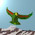## Wednesday, February 23, 2011

### Theory Update 66

Now let us write out $54$ trefoil quandles from the tetractys path set, graded according to the number of central neutrino paths in the quandle. The total number of each quandle type is noted in brackets.

zero(25)
$XXX,YYY,ZZZ$
$YYX,XXZ,ZZY$;$YXY,XZX,ZYZ$;$XYY,ZXX,YZZ$
$XXY,YYZ,ZZX$;$XYX,YZY,ZXZ$;$YXX,ZYY,XZZ$
$XXX,YYZ,ZZY$;$XXX,YZY,ZYZ$;$XXX,ZYY,YZZ$
$YYY,ZZX,XXZ$;$YYY,ZXZ,XZX$;$YYY,XZZ,ZXX$
$ZZZ,YYX,XXY$;$ZZZ,YXY,XYX$;$ZZZ,XYY,YXX$
$XXX,YYX,ZZX$;$XXX,YXY,ZXZ$;$XXX,XYY,XZZ$
$YYY,ZZY,XXY$;$YYY,ZYZ,XYX$;$YYY,YZZ,YXX$
$ZZZ,YYZ,XXZ$;$ZZZ,YZY,XZX$;$ZZZ,ZYY,ZXX$

three(2)
$XYZ,YZX,ZXY$;$XZY,ZYX,YXZ$

two(9)
$XYZ,YYY,ZYX$;$YZX,YYY,YXZ$;$ZXY,YYY,XZY$
$XZY,ZZZ,YZX$;$ZYX,ZZZ,ZXY$;$YXZ,ZZZ,XYZ$
$ZXY,XXX,YXZ$;$XYZ,XXX,XZY$;$YZX,XXX,ZYX$

one(18)
$XYZ,XZX,XXY$;$YZX,ZXX,XYX$;$ZXY,XXZ,YXX$
$XZY,XXZ,XYX$;$ZYX,XZX,YXX$;$YXZ,ZXX,XXY$
$XYZ,YYX,ZYY$;$YZX,YXY,YYZ$;$ZXY,XYY,YZY$
$XZY,ZYY,YXY$;$ZYX,YYZ,XYY$;$YXZ,YZY,YYX$
$XYZ,YZZ,ZXZ$;$YZX,ZZY,XZZ$;$ZXY,ZYZ,ZZX$
$XZY,ZZX,YZZ$;$ZYX,ZXZ,ZZY$;$YXZ,XZZ,ZYZ$

#### 3 comments:

1.The earthquake in Christchurch was top of the news last night (in Seattle), until this morning when we find out that two Seattle sailors were among those killed by pirates. Hope the city rebuilds stronger.

Meanwhile, a sleepless night left me convinced that I can do the renormalization on vertices. The secret is to assume that the vertices are built from extremely simple structures (i.e. MUBs). And then there are no vertices. This requires the force bosons to also be composite. And it makes the calculations easy to do on the computer.

2.This paper on electrical resistance of an infinite tiling of 1-ohm resistors: http://arxiv.org/abs/1102.4537 reminded me of the importance of the Fourier transform. I'm now appreciating the mathematical problem more, in the context of Hopf algebra.

I used the Fourier transform on 3x3 matrices to diagonalize the circulant matrices. After doing that, renormalization is easy: just keep the diagonal element which is largest. The others go to zero as you iterate. Then reverse transform to get the long time propagators (which are also attractors).

So my question is this: Is there a relationship between generalized Fourier transforms and the solutions to the renormalization process? I'm assuming necessary but not sufficient. That is, that any renormalization result is the inverse Fourier transform of an element of a basis set, or perhaps, a diagonal element.

3.Carl, for years now I have been working with twistor space techniques ... not the standard Feynman ones. This sounds like where you are heading. Generalized Fourier transforms are just one part of the big picture.

Note: Only a member of this blog may post a comment.